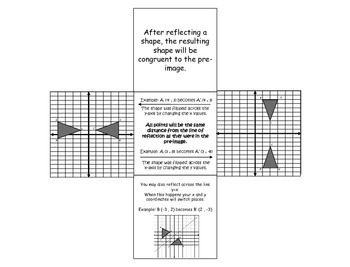digital

# 8th Grade Geometry Foldables ~Aligned to ALL 8th Grade CCSS Geometry StandardsSubject
Resource Type
Formats
PDF (2 MB)
TpT Digital Activity
Standards
\$4.00
TpT Digital Activity
Add notes & annotations through an interactive layer and assign to students via Google Classroom.
\$4.00
TpT Digital Activity
Add notes & annotations through an interactive layer and assign to students via Google Classroom.

### Description

This Geometry Foldables Bundle contains 11 Foldables and is aligned to the 8th Grade Common Core Geometry Unit.

This Bundle Includes:
8.G.1 & 8.G.2 – Types of Transformations Foldable (also shows the relation to similarity/congruence for each)
8.G.3 & 8.G.4 - Dilations Foldable (Includes an example on the coordinate plane and how each coordinate is effected)
8.G.3 & 8.G.4 - Reflections Foldable (Includes an example on the coordinate plane and how each coordinate is effected)
8.G.3 & 8.G.4 - Translations Foldable (Includes an example on the coordinate plane and how each coordinate is effected)
8.G.3 & 8.G.4 - Rotations Foldable (Includes an example on the coordinate plane and how each coordinate is effected)
8.G.5- Parallel Lines Cut By a Transversal Foldable (shows the many angle relationships that are formed)
8.G.5- Triangles Foldable (Includes what makes a triangle and types of triangles
8.G.6,7,8 – Pythagorean Theorem Foldable (Includes an example of why Pythagorean Theorem works, relevant vocabulary, how to find missing lengths using Pythagorean Theorem, common triples, and the Pythagorean Theorem Converse)
8.G.9- 3 Mini-Formulas Foldables (Includes area, volume, and surface area for various figures including spheres, cones, and cylinders)

***NEW- Directions (with pictures) for how to fold each product in the bundle!

Use these foldables to introduce new ideas or scaffold some of the more complex tasks that are associated with the Common Core Standards.
Total Pages
N/A
N/A
Teaching Duration
N/A
Report this Resource to TpT
Reported resources will be reviewed by our team. Report this resource to let us know if this resource violates TpT’s content guidelines.

### Standards

to see state-specific standards (only available in the US).
Know the formulas for the volumes of cones, cylinders, and spheres and use them to solve real-world and mathematical problems.
Apply the Pythagorean Theorem to find the distance between two points in a coordinate system.
Apply the Pythagorean Theorem to determine unknown side lengths in right triangles in real-world and mathematical problems in two and three dimensions.
Explain a proof of the Pythagorean Theorem and its converse.
Use informal arguments to establish facts about the angle sum and exterior angle of triangles, about the angles created when parallel lines are cut by a transversal, and the angle-angle criterion for similarity of triangles. For example, arrange three copies of the same triangle so that the sum of the three angles appears to form a line, and give an argument in terms of transversals why this is so.